Function Repository Resource:

# LaurentExtendedGCD

Compute the GCD and successive quotients for a pair of Laurent polynomials

Contributed by: Daniel Lichtblau
 ResourceFunction["LaurentExtendedGCD"][p1,p2,x] computes the extended GCD of the Laurent polynomials p1 and p2 in x.

## Details and Options

The polynomials must be univariate and have numeric coefficients.
The degree of a Laurent polynomial is the difference between the highest and lowest powers of the variable.
In contrast to the ring of ordinary polynomials, units are comprised of all monomials (since highest and lowest powers of the variable are the same).
A GCD for a pair of Laurent polynomials is unique up to unit factors.
Again in contrast to the case of ordinary polynomials, the list of quotients is not unique up to unit multiples. This is because we have the option of removing terms from either end in the process of taking a quotient.
ResourceFunction["LaurentExtendedGCD"] uses a method that attempts to keep coefficients as close to unity as possible in absolute value. The standard of proximity is relative; that is, it tries to minimize the maximum of the log of the coefficient absolute values. This is done via a greedy approach: nontrivial reductions typically allow either two or three possible quotients (reduce two coefficients at the top, two at the bottom, or one at each end, if degrees differ by one). The best one at each step is chosen by the criterion above.
ResourceFunction["LaurentExtendedGCD"] takes a Tolerance option. With the setting Tolerancetol, an inexact numeric coefficient c is considered to be zero if Chop[c,tol] is zero.
The tolerance must be a non-negative real value.
When the coefficients are approximate numbers, the default tolerance is 10-8.
The tolerance can also be specified as a pair {tol1,tol2}. In this case tol1 is used as above while tol2 is a relative tolerance. In particular, if the coefficient list of a polynomial is clist, then Chop[clist,Max[Abs[clist]]tol] is also performed.
An important application of Laurent extended GCD is in wavelet computations, where the quotient sequence is used to create fast lifting filters.
In the wavelet setting one can also obtain a set of valid quotients and remainder using WaveletFilterCoefficients; it need not (and in general will not) be the same as the set given by ResourceFunction["LaurentExtendedGCD"].

## Examples

### Basic Examples (2)

Compute a quotient sequence and GCD for two polynomials:

 In:=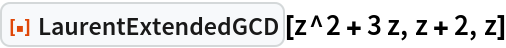Out=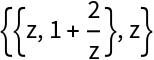Create a pair of random Laurent polynomials of degree 10:

 In:=Out=Compute a quotient sequence and GCD:

 In:=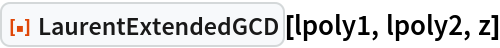Out=### Scope (2)

Create two exact Laurent polynomials:

 In:=Out=Compute a quotient sequence and GCD:

 In:=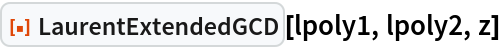Out=LaurentExtendedGCD can return a nontrivial GCD:

 In:=Out=Compute a quotient sequence and GCD:

 In:=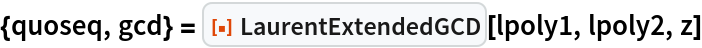Out=LaurentExtendedGCD works with approximate numeric coefficients and gives an equivalent result:

 In:=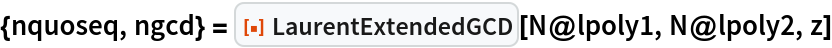Out=Compare the difference numerically:

 In:=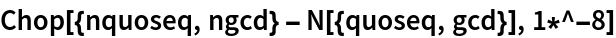Out=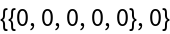### Properties and Relations (23)

Form the Laurent pair corresponding to the Daubechies 6 (or db3) wavelet filter coefficients:

 In:=Out=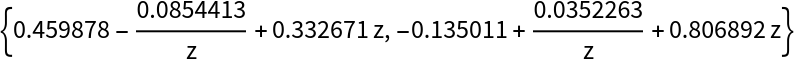Compute the GCD and quotients:

 In:=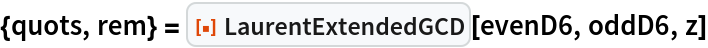Out=A set of quotients and remainder can also be obtained using WaveletFilterCoefficients with "LiftingFilter" and "LiftingLaurentForm":

 In:=Out=#### The Daubechies4 wavelet and the forward lifting transform (23)

Obtain the Daubechies 4 (D4, also called db2) wavelet coefficients:

 In:=Out=Create two corresponding Laurent polynomials:

 In:=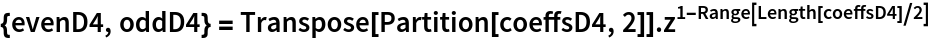Out=Compute the Laurent GCD and list of quotients:

 In:=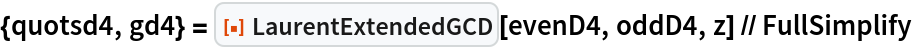Out=Create a list of 2×2 matrices that represent the operation of reducing a pair of Laurent polynomials by their quotient:

 In:=Out=Take their product:

 In:=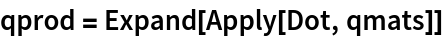Out=Applying this product to the vector comprised of the GCD and 0 recovers the original pair of Laurent polynomials:

 In:=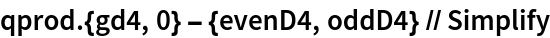Out=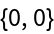Invert the reducing matrices:

 In:=Out=Form the reverse-product of these inverses:

 In:=Out=Check that this is in fact the inverse:

 In:=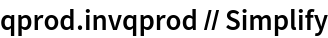Out=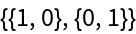This inverse product matrix provides the Bezout multipliers (top row) and syzygy (bottom row), as can be seen by its action on the input pair:

 In:=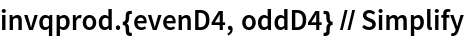Out=Create the quadrature mirror filter from the D4 coefficients:

 In:=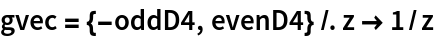Out=A certain upper triangular matrix is needed to recover these Laurent polynomials:

 In:=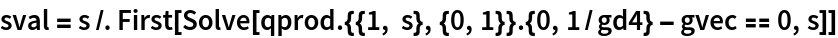Out=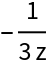Form the augmented set of 2×2 matrices required to create the wavelet polyphase matrix:

 In:=Out=Compute the polyphase matrix:

 In:=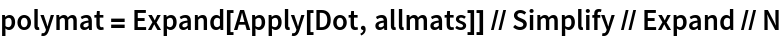Out=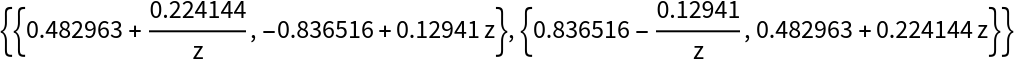Show that the second column is indeed the quadrature mirror filter:

 In:=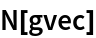Out=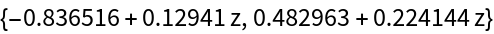The same matrix can be obtained from WaveletFilterCoefficients:

 In:=Out=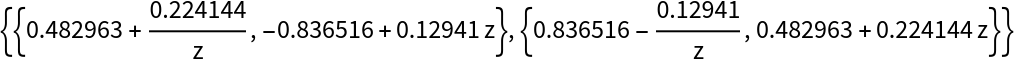Create a function to recover the discrete wavelet transform (DWT) from these 2×2 matrix factors. For this, powers of the Laurent variable z become shifts with positive exponents being leftward, and indices are modulo the length of the sequence (that is, using periodic wrapping):

 In:=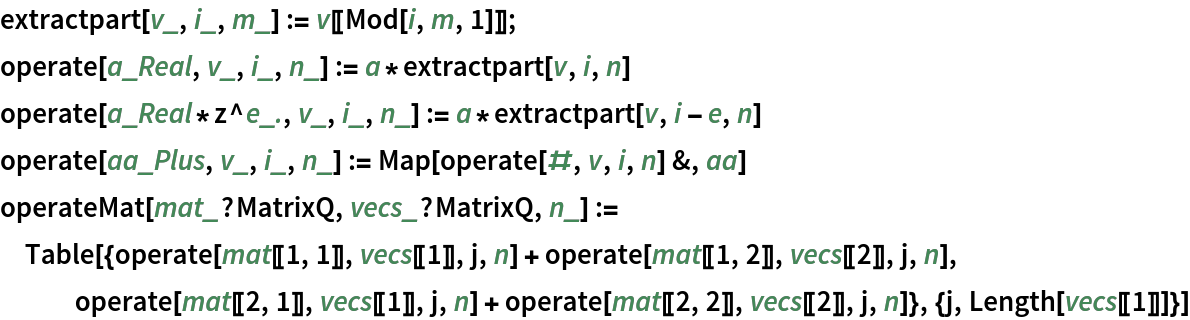Work with {1,2,,16} split into odds and evens (the so-called "Lazy Wavelet Transform"):

 In:=Compute the DWT:

 In:=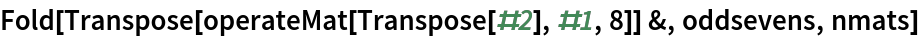Out=Compare to the forward lift from the LiftingFilterData for the D2 wavelet:

 In:=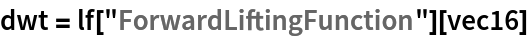Out=Get the same result directly from LiftingWaveletTransform:

 In:=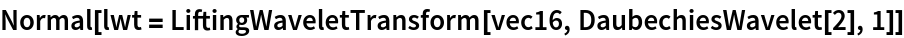Out=Invert the process to recover the original values, using Riffle at the end to undo the Lazy Wavelet Transform:

 In:=Out=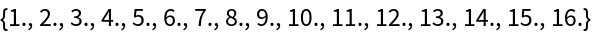This is equivalent to using InverseWaveletTransform on the DiscreteWaveletData object:

 In:=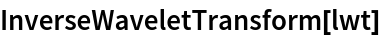Out=The conventional way to use the quotient matrices and their inverses to form a lifting filter actually makes them alternating upper and lower triangular. For simplicity of exposition, this step was omitted.

### Neat Examples (12)

#### Compressing an image with the Daubechies10 wavelet (12)

Create the even and odd Laurent polynomials that arise from the Daubechies10 wavelet coefficients:

 In:=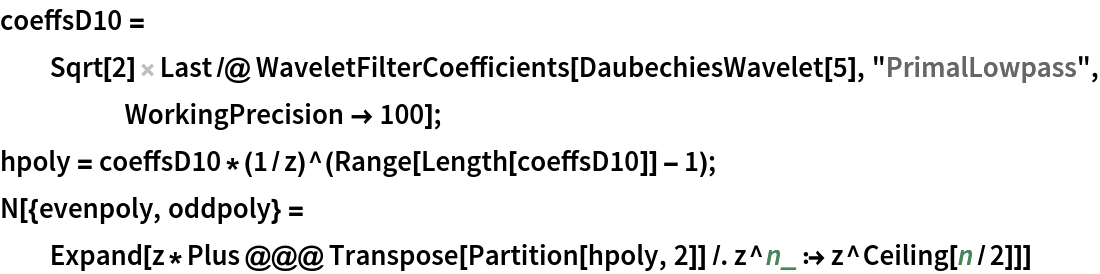Out=Compute an extended GCD with quotients coefficients that are reasonably close in magnitude to unity:

 In:=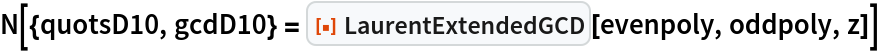Out=Another good set of quotients for the D10 Laurent pair is given in the reference by Maslen and Abbott; the latter happens to be the one provided by WaveletFilterCoefficients:

 In:=Out=Create the quotient matrices and the scaling value (a factor of z2 is required for this particular factorization):

 In:=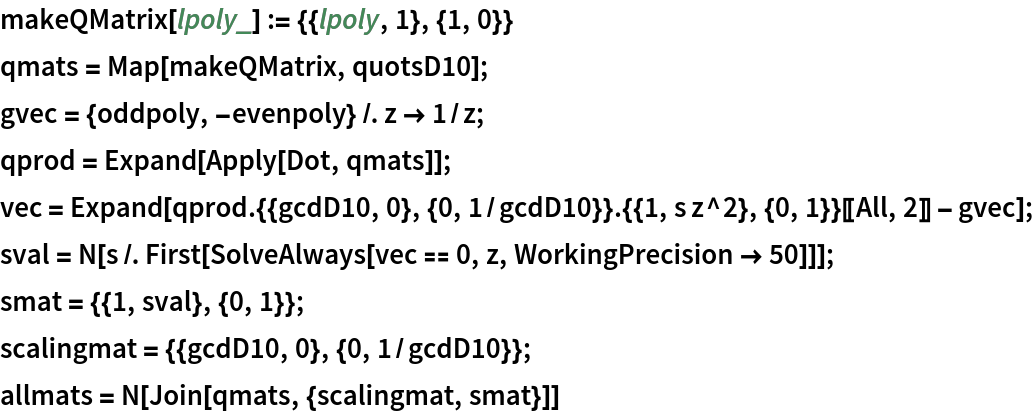Out=Set up functions that transform from Laurent polynomials to vector operations:

 In:=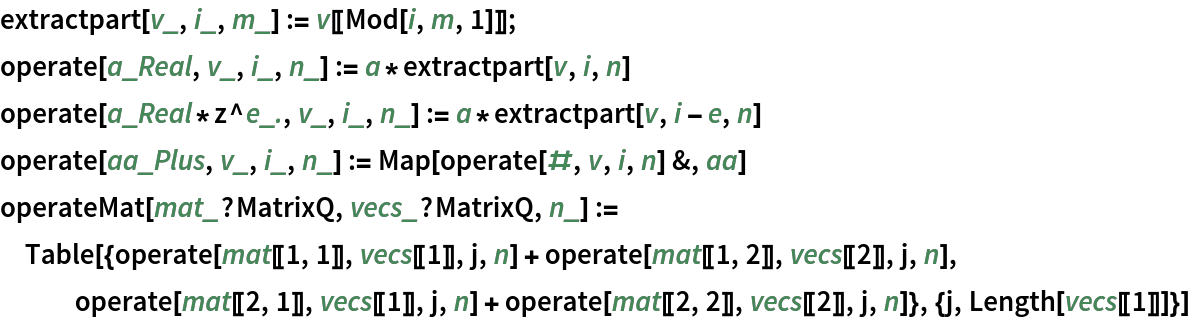Pad a square image to have a power of 2 dimension, removing the average pixel value from each row:

 In:=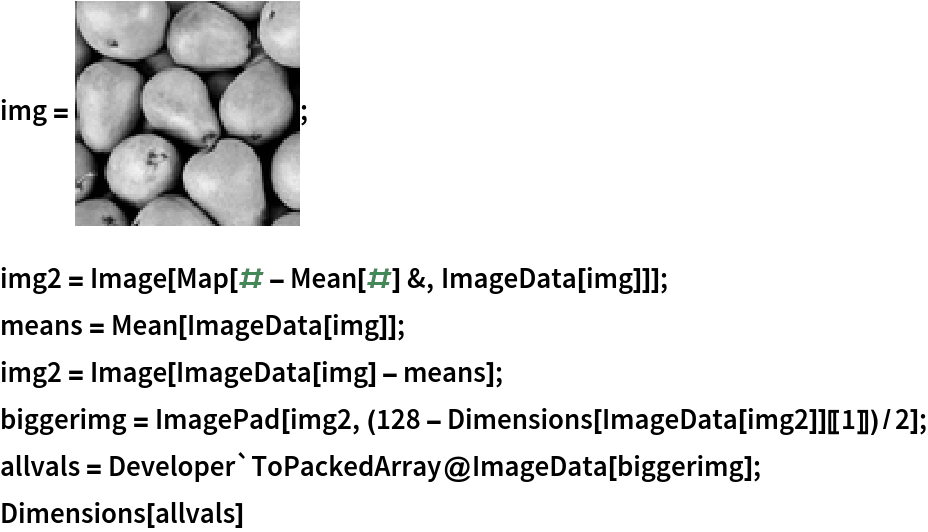Out=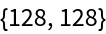Compute the DWT on rows and then compute the DWT on the columns of that result:

 In:=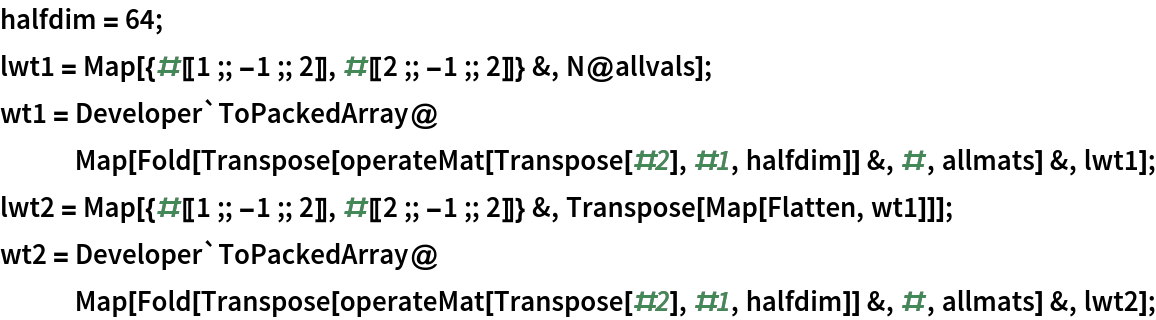Create a sparse array, chopping values larger than 0.1 to reduce size:

 In:=Out=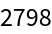Create the inverse operations:

 In:=Perform one inversion and compare to the first level of the previous discrete transform:

 In:=Out=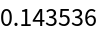Perform a second inversion and compare to the input:

 In:=Out=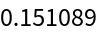Recover a reasonable approximation to the original image:

 In:=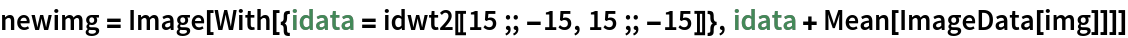Out=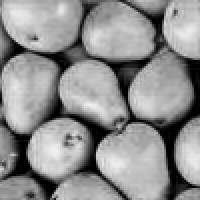## Version History

• 1.0.0 – 08 February 2022